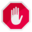## Name

ST_CollectionExtract — Given a geometry collection, returns a multi-geometry containing only elements of a specified type.

## Synopsis

`geometry ST_CollectionExtract(`geometry collection`)`;

`geometry ST_CollectionExtract(`geometry collection, integer type`)`;

## Description

Given a geometry collection, returns a homogeneous multi-geometry.

If the `type` is not specified, returns a multi-geometry containing only geometries of the highest dimension. So polygons are preferred over lines, which are preferred over points.

If the `type` is specified, returns a multi-geometry containing only that type. If there are no sub-geometries of the right type, an EMPTY geometry is returned. Only points, lines and polygons are supported. The type numbers are:

• 1 == POINT

• 2 == LINESTRING

• 3 == POLYGON

For atomic geometry inputs, the geometry is retured unchanged if the input type matches the requested type. Otherwise, the result is an EMPTY geometry of the specified type. If required, these can be converted to multi-geometries using ST_Multi.MultiPolygon results are not checked for validity. If the polygon components are adjacent or overlapping the result will be invalid. (For example, this can occur when applying this function to an ST_Split result.) This situation can be checked with ST_IsValid and repaired with ST_MakeValid.

Availability: 1.5.0Prior to 1.5.3 this function returned atomic inputs unchanged, no matter type. In 1.5.3 non-matching single geometries returned a NULL result. In 2.0.0 non-matching single geometries return an EMPTY result of the requested type.

## Examples

Extract highest-dimension type:

```SELECT ST_AsText(ST_CollectionExtract(
'GEOMETRYCOLLECTION( POINT(0 0), LINESTRING(1 1, 2 2) )'));
st_astext
---------------
MULTILINESTRING((1 1, 2 2))
```

Extract points (type 1 == POINT):

```SELECT ST_AsText(ST_CollectionExtract(
'GEOMETRYCOLLECTION(GEOMETRYCOLLECTION(POINT(0 0)))',
1 ));
st_astext
---------------
MULTIPOINT((0 0))
```

Extract lines (type 2 == LINESTRING):

```SELECT ST_AsText(ST_CollectionExtract(
'GEOMETRYCOLLECTION(GEOMETRYCOLLECTION(LINESTRING(0 0, 1 1)),LINESTRING(2 2, 3 3))',
2 ));
st_astext
---------------
MULTILINESTRING((0 0, 1 1), (2 2, 3 3))
```